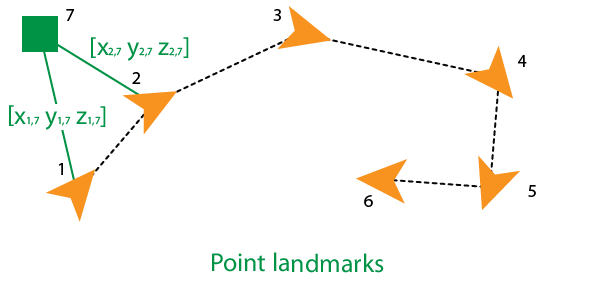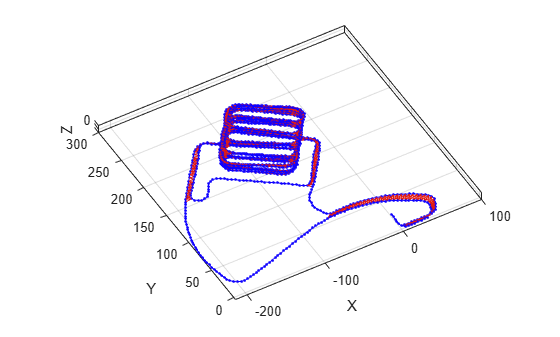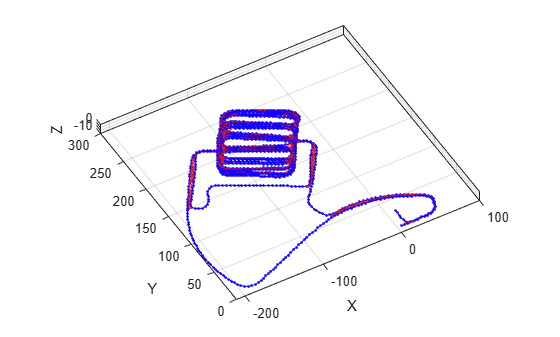Main Content

# poseGraph3D

Create 3-D pose graph

## Description

A `poseGraph3D` object stores information for a 3-D pose graph representation. A pose graph contains nodes connected by edges. Each node estimate is connected to the graph by edge constraints that define the relative pose between nodes and the uncertainty on that measurement.

To construct a pose graph iteratively, use the `addRelativePose` function to add relative pose estimates and connect them to an existing node with specified edge constraints. Pose nodes must be specified relative to a pose node. Specify the uncertainty of the measurement using an information matrix.Adding an edge between two nonsequential nodes creates a loop closure in the graph. Multiple edges or multiedges between node pairs are also supported, which includes loop closures. To add additional edge constraints or loop closures, specify the node IDs using the `addRelativePose` function. When optimizing the pose graph, the `optimizePoseGraph` function finds a solution to satisfy all these edge constraints.To add landmark point nodes, use the `addPointLandmark` function. This function specifies nodes as xyz-points without orientation estimates. Landmarks must be specified relative to a pose node.For 2-D pose graphs, see `poseGraph`.

For an example that builds and optimizes a 3-D pose graph from real-world sensor data, see Landmark SLAM Using AprilTag Markers.

## Creation

### Syntax

``poseGraph = poseGraph3D``
``poseGraph = poseGraph3D('MaxNumEdges',maxEdges,'MaxNumNodes',maxNodes)``

### Description

example

``` `poseGraph = poseGraph3D` creates a 3-D pose graph object. Add poses using `addRelativePose` to construct a pose graph iteratively.```
``` `poseGraph = poseGraph3D('MaxNumEdges',maxEdges,'MaxNumNodes',maxNodes)` specifies an upper bound on the number of edges and nodes allowed in the pose graph when generating code. This limit is only required when generating code.```

## Properties

expand all

This property is read-only.

Number of nodes in pose graph, specified as a positive integer. Each node represents a pose measurement or a point landmark measurement. To specify relative poses between nodes, use `addRelativePose`. To specify a landmark pose, use `addLandmarkPose`. To get a list of all nodes, use `edgeNodePairs`.

This property is read-only.

Number of edges in pose graph, specified as a nonnegative integer. Each edge connects two nodes in the pose graph. Loop closure edges and landmark edges are included.

This property is read-only.

Number of loop closures in pose graph, specified as a nonnegative integer. To get the edge IDs of the loop closures, use the `LoopClosureEdgeIDs` property.

This property is read-only.

Loop closure edges IDs, specified as a vector of edge IDs.

This property is read-only.

Landmark node IDs, specified as a vector of IDs for each node.

## Object Functions

 `addPointLandmark` Add landmark point node to pose graph `addRelativePose` Add relative pose to pose graph `copy` Create copy of pose graph `edgeNodePairs` Edge node pairs in pose graph `edgeConstraints` Edge constraints in pose graph `edgeResidualErrors` Compute pose graph edge residual errors `findEdgeID` Find edge ID of edge `nodeEstimates` Poses of nodes in pose graph `removeEdges` Remove loop closure edges from graph `show` Plot pose graph

## Examples

collapse all

Optimize a pose graph based on the nodes and edge constraints. The pose graph used in this example is taken from the MIT Dataset and was generated using information extracted from a parking garage.

Load the pose graph from the MIT dataset. Inspect the `poseGraph3D` object to view the number of nodes and loop closures.

```load parking-garage-posegraph.mat pg disp(pg);```
``` poseGraph3D with properties: NumNodes: 1661 NumEdges: 6275 NumLoopClosureEdges: 4615 LoopClosureEdgeIDs: [1x4615 double] LandmarkNodeIDs: [1x0 double] ```

Plot the pose graph with IDs off. Red lines indicate loop closures identified in the dataset.

```title('Original Pose Graph') show(pg,'IDs','off'); view(-30,45)```Optimize the pose graph. Nodes are adjusted based on the edge constraints and loop closures. Plot the optimized pose graph to see the adjustment of the nodes with loop closures.

```updatedPG = optimizePoseGraph(pg); figure title('Updated Pose Graph') show(updatedPG,'IDs','off'); view(-30,45)```Carlone, Luca, Roberto Tron, Kostas Daniilidis, and Frank Dellaert. "Initialization Techniques for 3D SLAM: a Survey on Rotation Estimation and its Use in Pose Graph Optimization." 2015 IEEE International Conference on Robotics and Automation (ICRA). 2015, pp. 4597–4604.